Filters
Size

# Archive Items

Filters

Selected Filters• XS
• S
• M
• L
• XL
• XXL
• Select Size
Sold Out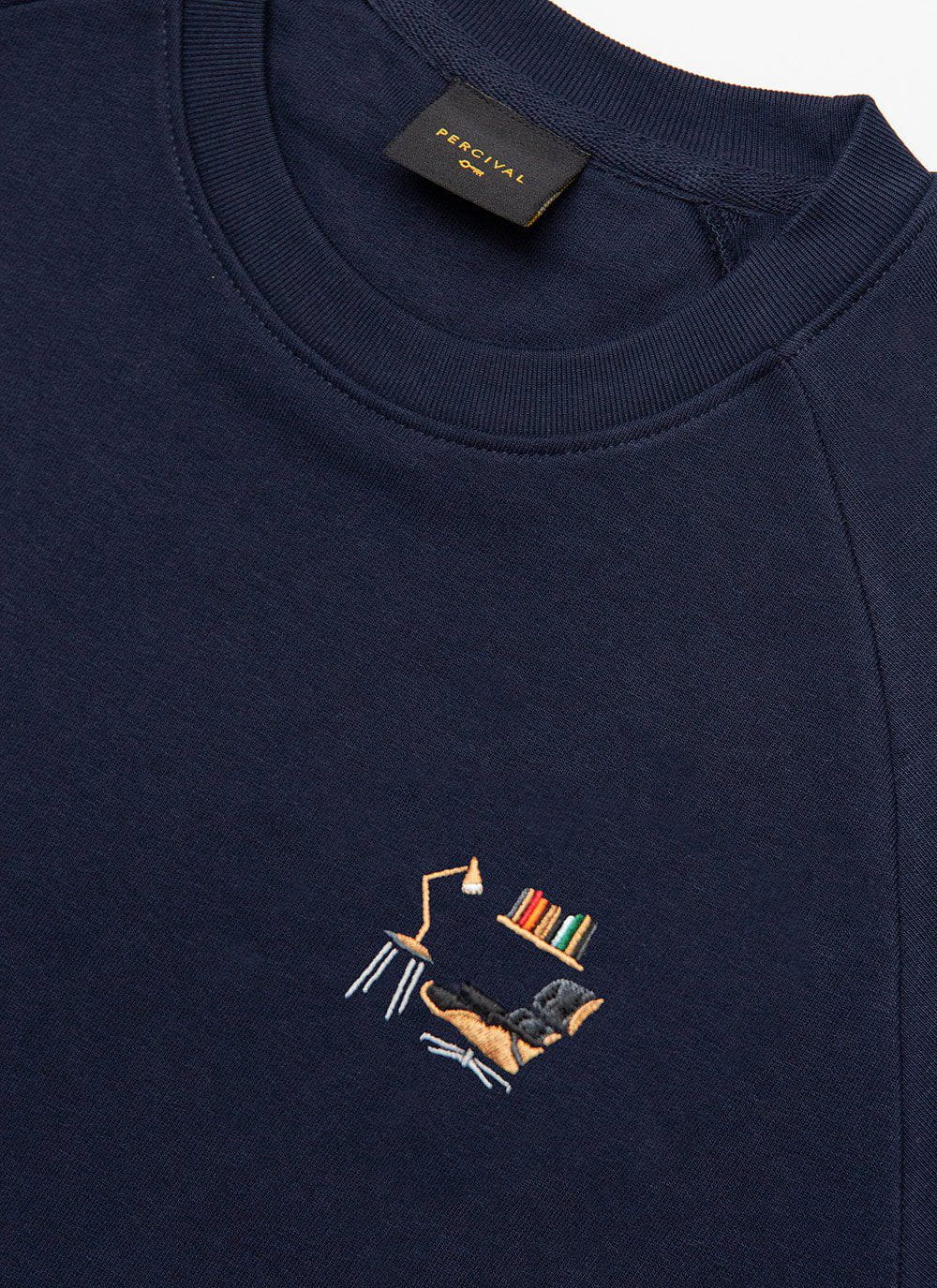• XS
• S
• M
• L
• XL
• XXL
• Select Size
Running Low• M
• L
• XL
• Select Size
Sold Out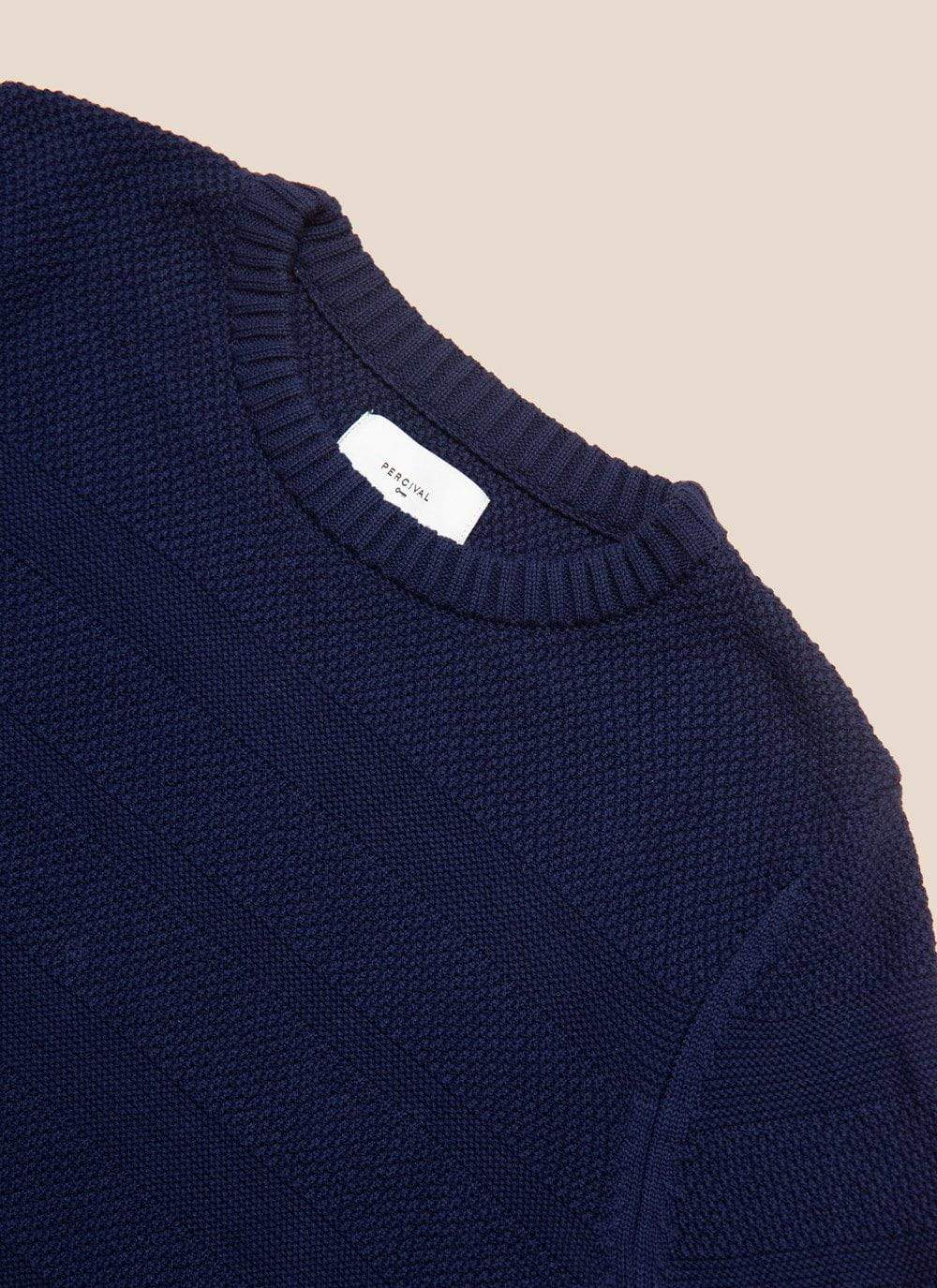• XS
• S
• M
• L
• XL
• XXL
• Select Size
Sold Out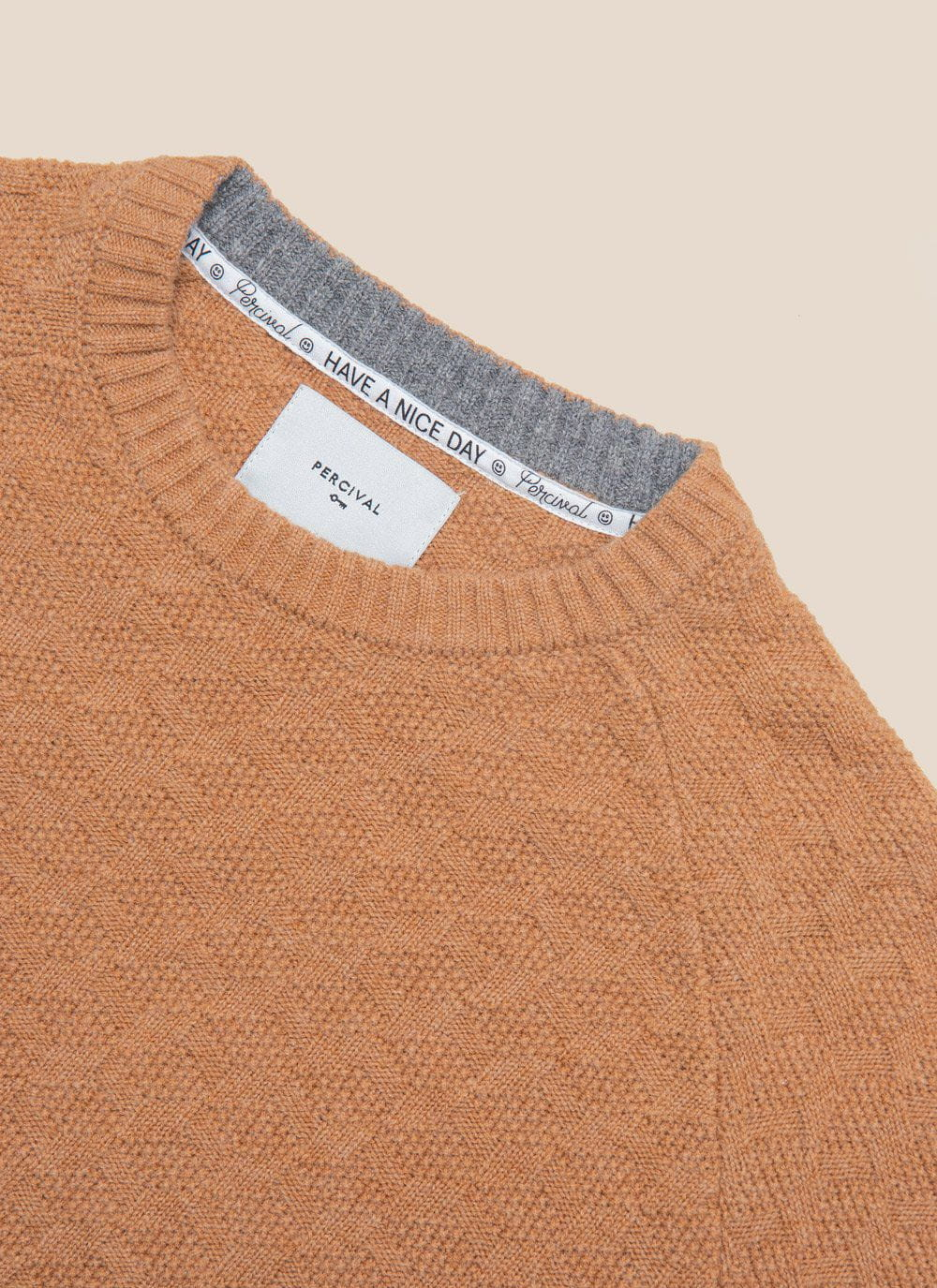• XS
• S
• M
• L
• XL
• XXL
• Select Size
Running Low• M
• L
• XL
• Select Size• M
• L
• XL
• Select Size
Sold Out• XS
• S
• M
• L
• XL
• XXL
• XXXL
• Select Size
Sold Out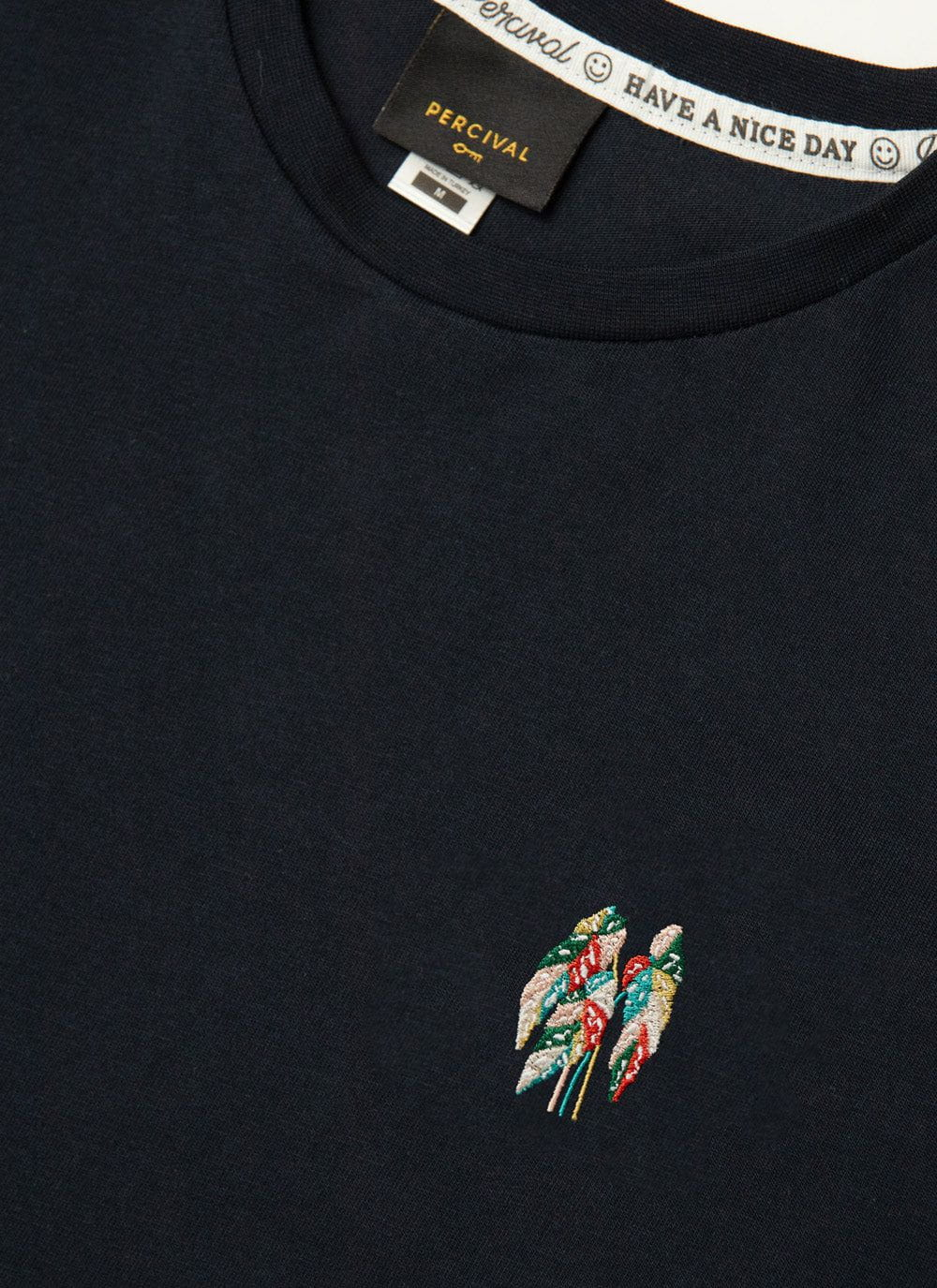• XS
• S
• M
• L
• XL
• XXL
• Select Size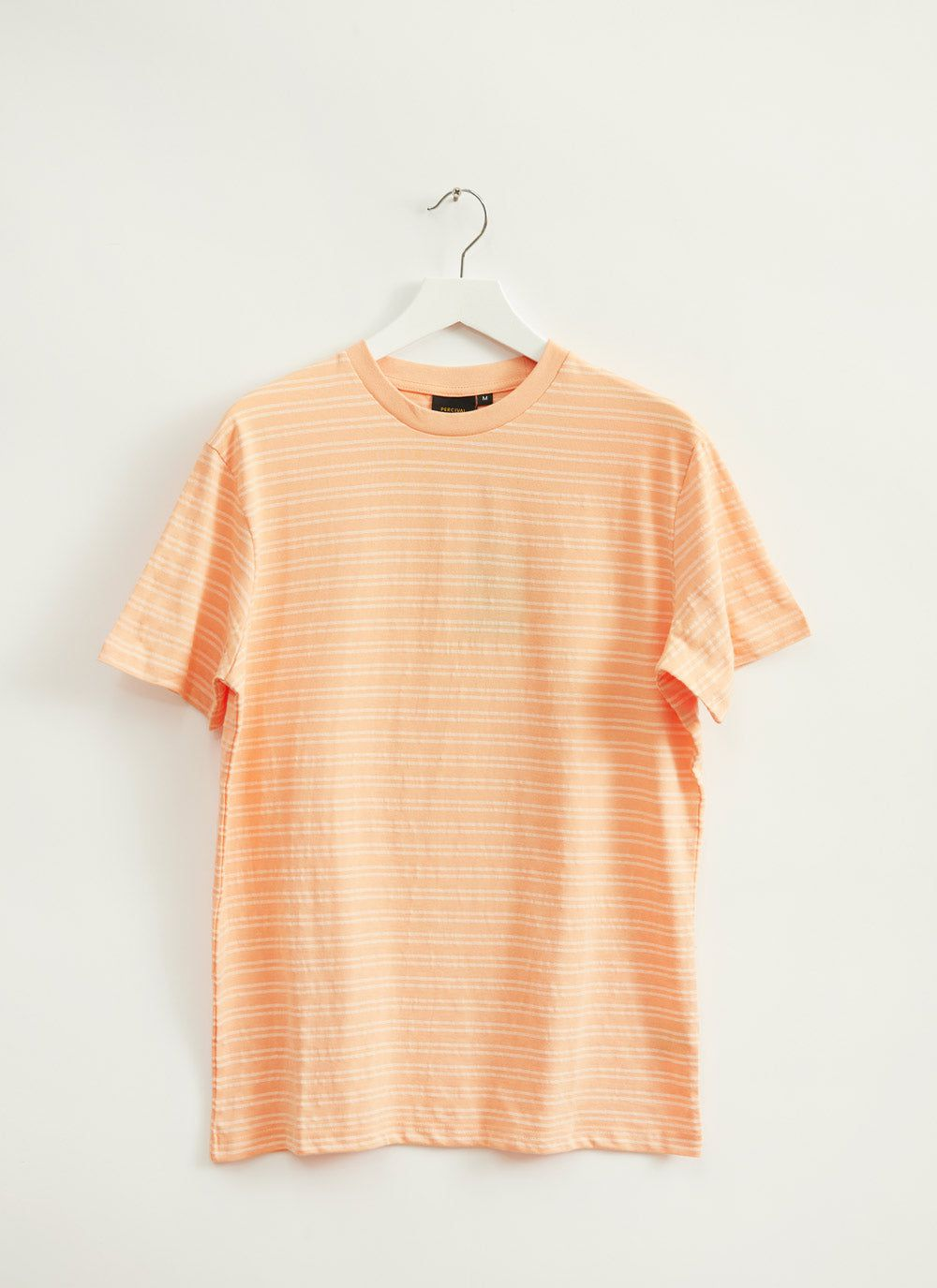• S
• M
• L
• XL
• Select Size
Sold Out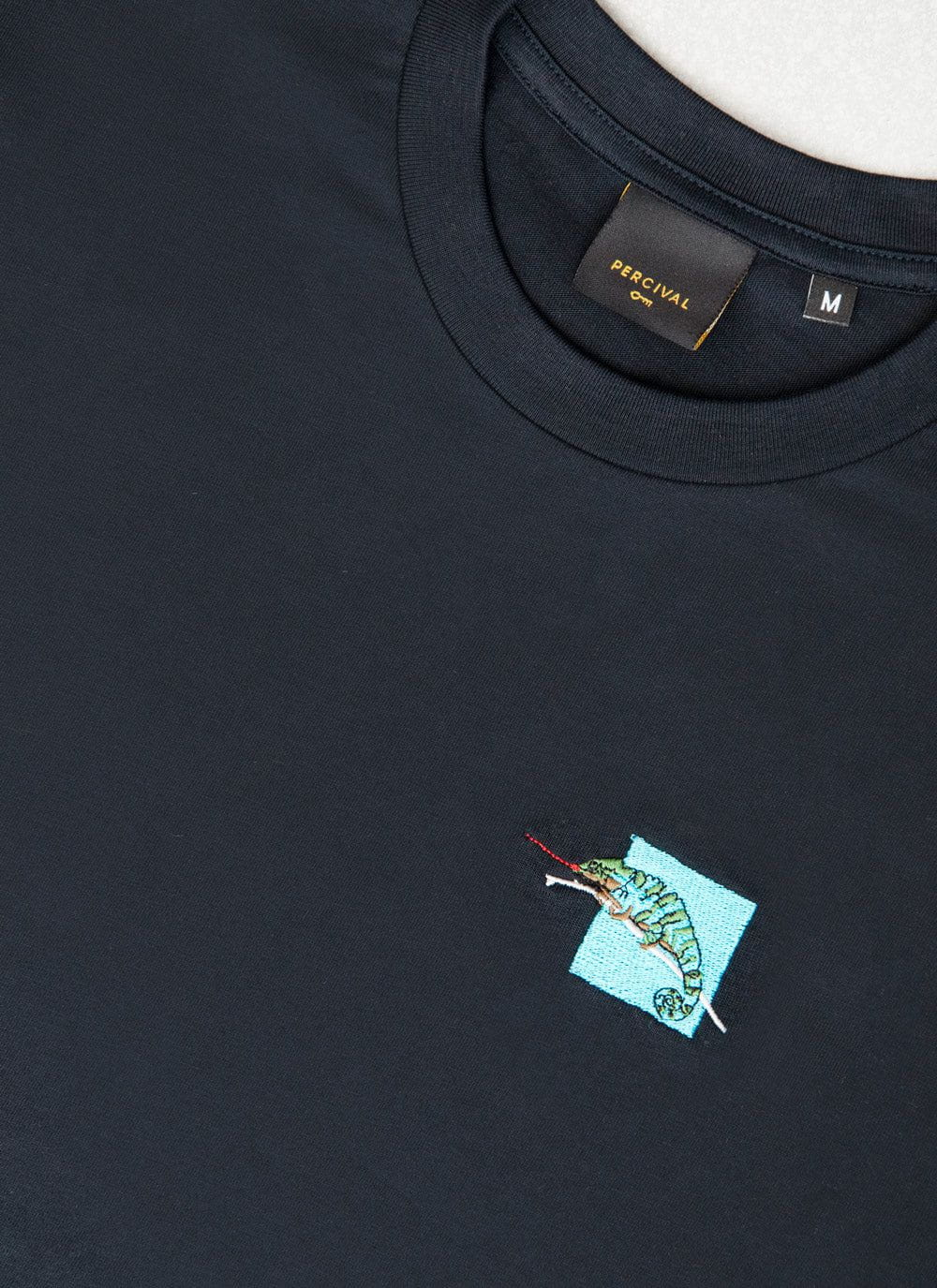• XS
• S
• M
• L
• XL
• XXL
• Select Size
Sold Out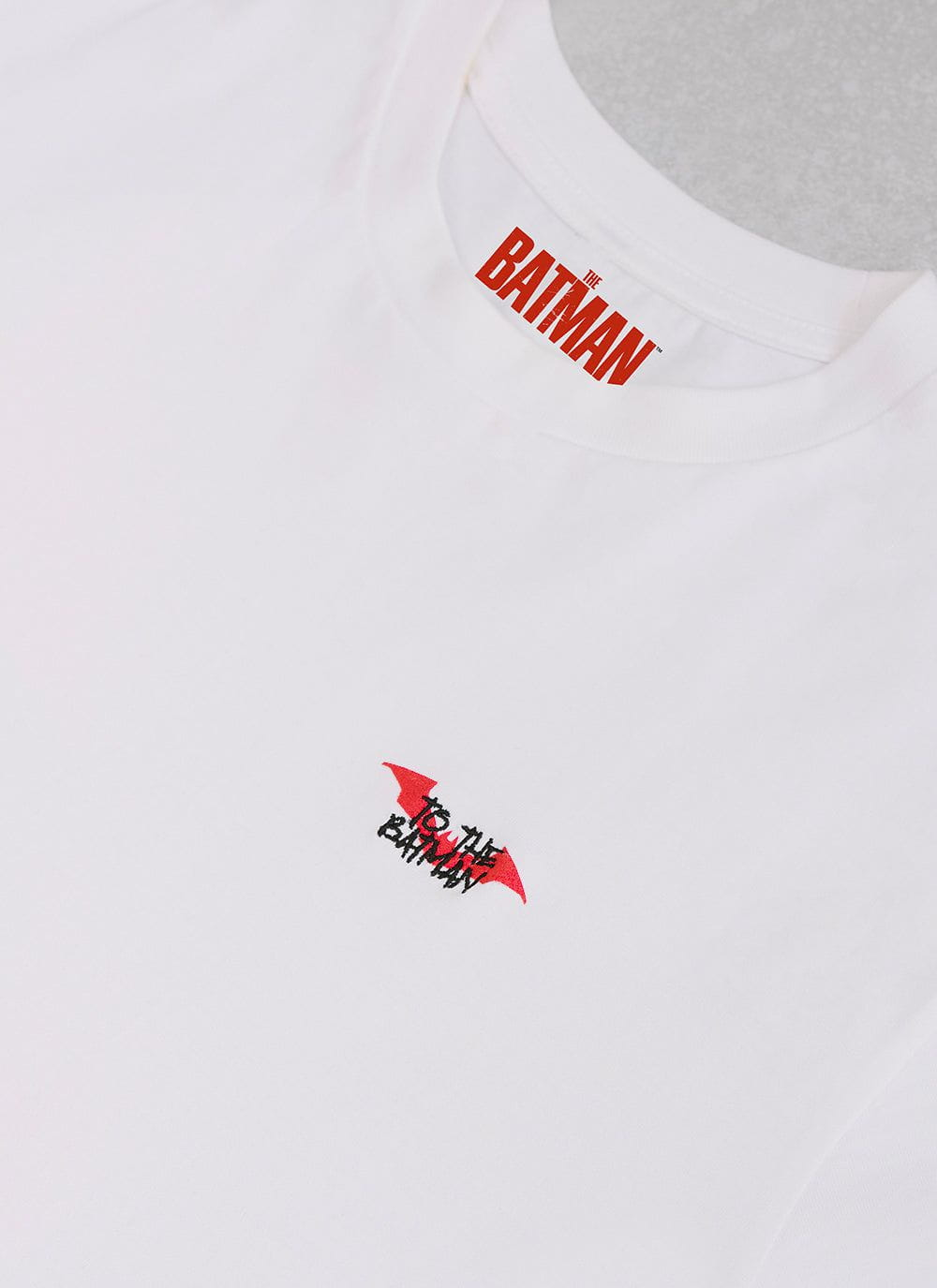• XS
• S
• M
• L
• XL
• XXL
• Select Size